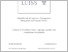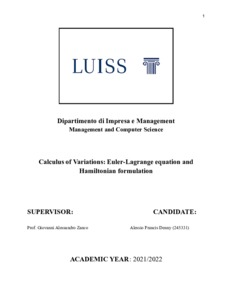# LuissThesis

## Calculus of variations: Euler–Lagrange equation and Hamiltonian formulation

Denny, Alessio Francis (A.A. 2021/2022) Calculus of variations: Euler–Lagrange equation and Hamiltonian formulation. Tesi di Laurea in Mathematics, Luiss Guido Carli, relatore Giovanni Alessandro Zanco, pp. 109. [Bachelor's Degree Thesis]Preview
PDF (Full text)

## Abstract/Index

Background theory of differential equations. Picard-Lindelöf general statement of the theorem. Intuition behind Cauchy sequences. Intuition behind Cauchy sequences of functions, pointwise convergence and uniform convergence. Complete proof of the Picard-Lindelöf theorem. Optimization intuition. First and second variation of a functional. The Euler-Lagrange equation derivation and intuition. Special cases of the Euler-Lagrange differential equation. The brachistochrone problem. The Hamiltonian. Integral, non-integral constraints and variable end-point problems.

## References

Bibliografia: pp. 107-108.

Thesis Type: Bachelor's Degree Thesis Luiss Guido Carli Bachelor's Degree Programs > Bachelor's Degree Program in Management and Computer Science, English language (L-18) Mathematics Zanco, Giovanni Alessandro 2021/2022 Summer Alessandro Perfetti 03 Nov 2022 15:34 03 Nov 2022 15:34 https://tesi.luiss.it/id/eprint/33771View Item